# Properties of Parallelograms

 1 What is a Parallelogram? 2 Properties of a Quadrilateral 3 Angles of a Parallelogram 4 Diagonals of a Parallelogram 5 Properties of a Parallelogram 6 Opposite Sides of a Parallelogram are Equal 7 Opposite Angles of a Parallelogram are Equal 8 Diagonals Bisect each other in a Parallelogram 9 One Pair of Opposite Sides is Equal and Parallel in a Parallelogram 10 Important Notes 11 Solved Examples 12 Thinking out of the box! 13 Maths Olympiad Sample Papers 14 Frequently Asked Questions (FAQs) 15 Download FREE Worksheets

## What is a Parallelogram?

A parallelogram is a convex polygon with 4 edges and 4 vertices.

It is a type of quadrilateral in which the opposite sides are parallel and equal.

Drag the vertices to understand the relation between the different elements of a parallelogram.

• A quadrilateral is a closed shape with 4 sides.
• All the internal angles of a quadrilateral add up to 360°

## Angles of a Parallelogram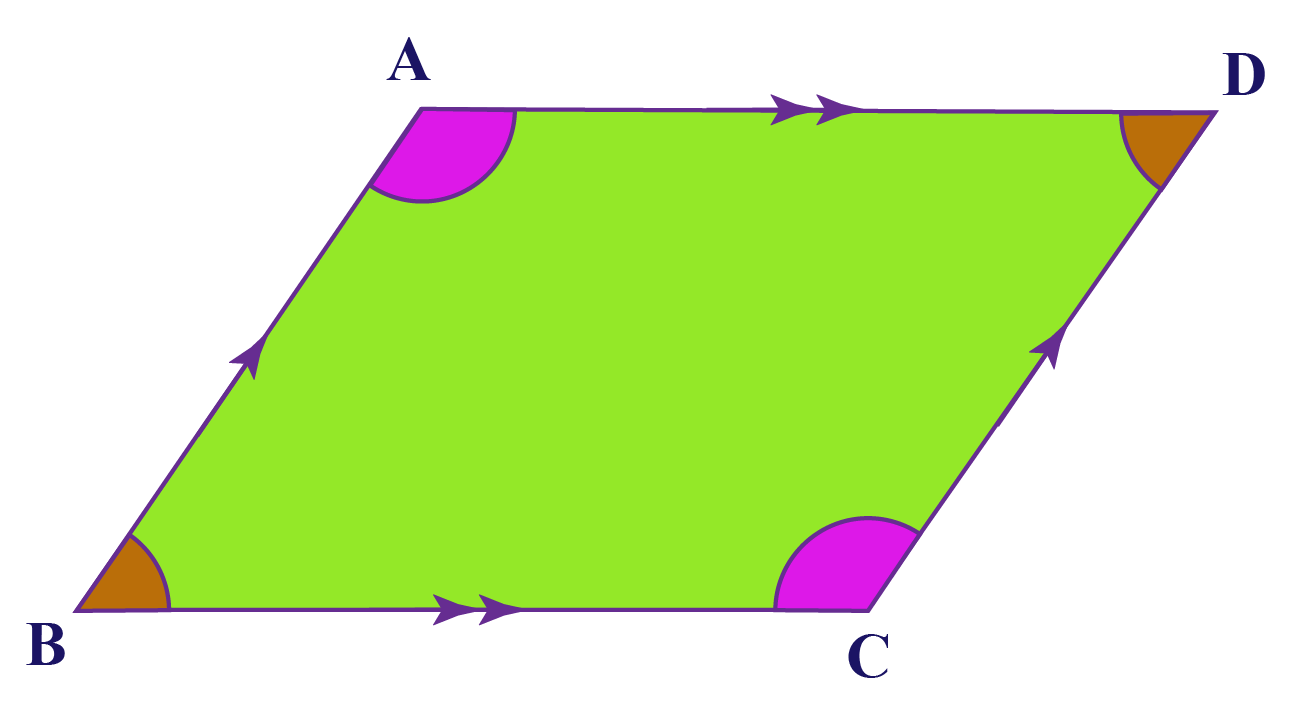The angles of a parallelogram are the 4 angles formed at the vertices.

• They all add up to 360$$^\circ$$
($$\angle A +\angle B +\angle C +\angle D = 360^\circ$$)

• Opposite angles are equal
($$\angle A = \angle C\: \text{and} \:\angle B = \angle D$$)

• Consecutive angles are supplementary
• $$\angle A +\angle B= 180^\circ$$
• $$\angle B +\angle C= 180^\circ$$
• $$\angle C +\angle D= 180^\circ$$
• $$\angle A +\angle D= 180^\circ$$

## Diagonals of a Parallelogram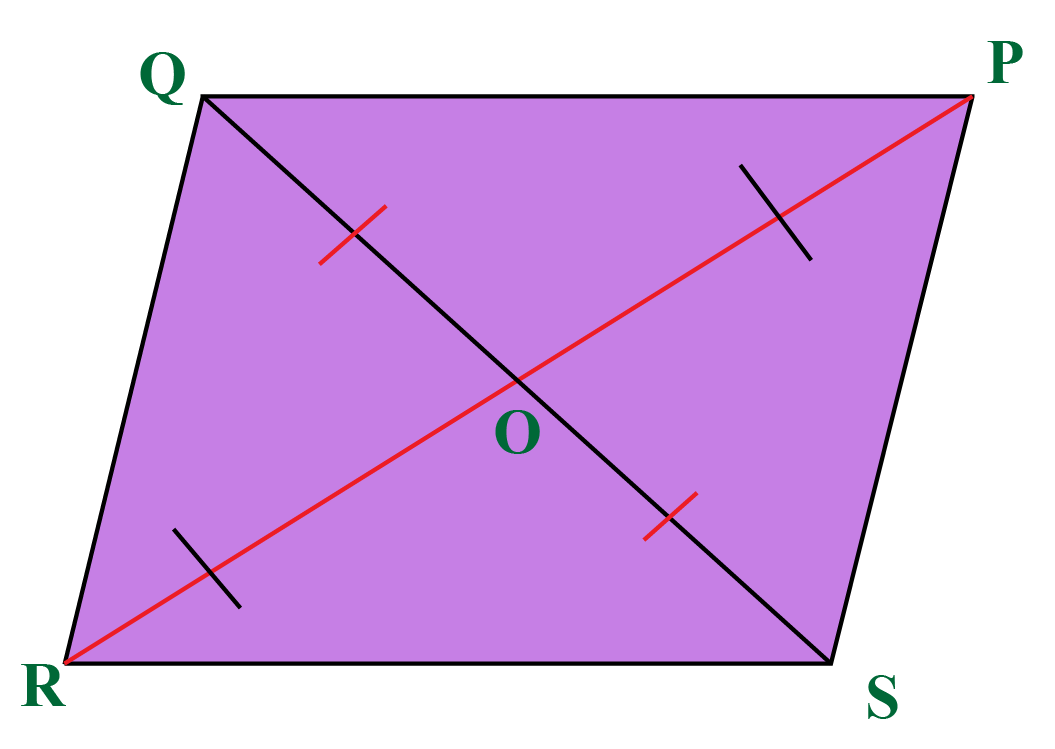Diagonals are line segments which join the opposite vertices.

In parallelogram $$PQRS$$, $$PR$$ and $$QS$$ are the diagonals.

• Diagonals bisect each other
$$OQ =OS$$  and $$OR = OP$$

• Each diagonal divides the parallelogram into two congruent triangles.
$$\Delta RSP\cong PQR$$
$$\Delta QPS\cong SRQ$$

• Parallelogram Law: The sum of the squares of the sides is equal to the sum of the squares of the diagonals.

## What are the 4 Properties of a Parallelogram?

### Theorems related to Parallelogram

The definition of a parallelogram is that it is a quadrilateral in which the opposite sides are parallel.

From this definition, we will show that:

1. The opposite sides of a parallelogram are equal (and conversely: if the opposite sides of a quadrilateral are equal, it is a parallelogram)
2. The opposite angles of a parallelogram are equal (and conversely: if the opposite angles of a quadrilateral are equal, it is a parallelogram)
3. The diagonals of a parallelogram bisect each other (and conversely: if the diagonals of a quadrilateral bisect each other, it is a parallelogram)
4. If one pair of opposite sides of a quadrilateral is equal and parallel, the quadrilateral is a parallelogram

## Opposite Sides of a Parallelogram are Equal

In a parallelogram, opposite sides are equal.

Conversely, if the opposite sides in a quadrilateral are equal, then it is a parallelogram.

Consider the following figure: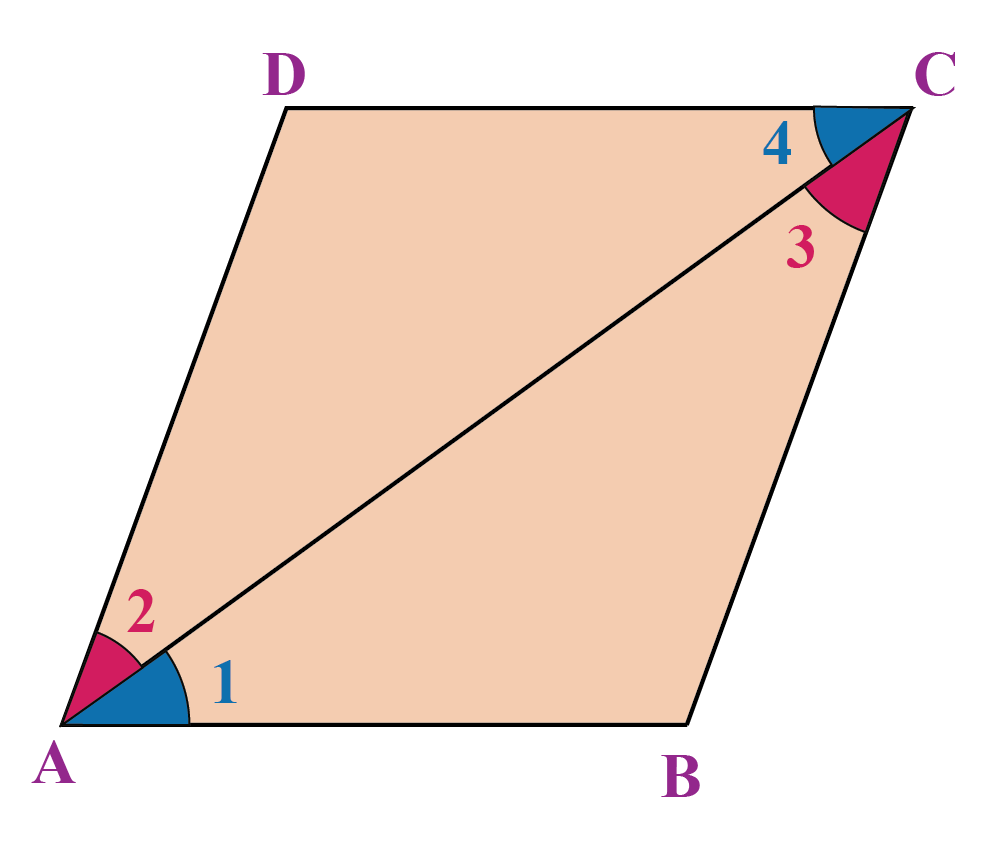### Proof:

We will assume that $$ABCD$$ is a parallelogram.

Compare $$\Delta ABC$$ and $$\Delta CDA$$:

\begin{align} & AC=CA \\ &\left( \text{common sides}\right)\\\\ & \angle 1=\angle 4\\ &\left( \text{alternate}\ \text{interior}\ \text{angles} \right)\\\\ & \angle 2=\angle 3 \\ &\left( \text{alternate}\ \text{interior}\ \text{angles} \right) \end{align}

Thus, by the ASA criterion, the two triangles are congruent, which means that the corresponding sides must be equal.

Thus,

\begin{align}\boxed{AB=CD\;\text{and}\;AD=BC} \end{align}

Now, we will prove the converse of this.

### Converse of the Theorem

If the opposite sides in a quadrilateral are equal, then it is a parallelogram.

Assume that $$ABCD$$ is a quadrilateral in which $$AB = CD$$  and $$AD = BC$$,

Compare $$\Delta ABC$$ and $$\Delta CDA$$ once again:

\begin{align} & AC=AC\\ &\left( \text{common sides}\right) \\\\ & AB=CD \\ &\left( \text{since alternate interior angles are equal } \right)\\\\ & AD=BC \\ &\left( \text{given}\right) \end{align}

\begin{align} & AC=AC\\ &\left( \text{common sides}\right) \\\\ & AB=CD \\ &\left( \text{since alternate interior}\\ \text{angles are equal } \right)\\\\ & AD=BC \\ &\left( \text{given}\right) \end{align}

Thus, by the SSS criterion, the two triangles are congruent, which means that the corresponding angles are equal:

\begin{align} & \angle 1=\angle 4\Rightarrow AB\parallel CD\ \\ & \angle 2=\angle 3\Rightarrow AD\parallel BC\ \end{align}

Hence,

\begin{align}\boxed{ AB\parallel CD\;\text{and}\;AD\parallel BC}\end{align}

Thus, $$ABCD$$ is a parallelogram.

## Opposite Angles of a Parallelogram are Equal

In a parallelogram, opposite angles are equal.

Conversely, if the opposite angles in a quadrilateral are equal, then it is a parallelogram.

Consider the following figure: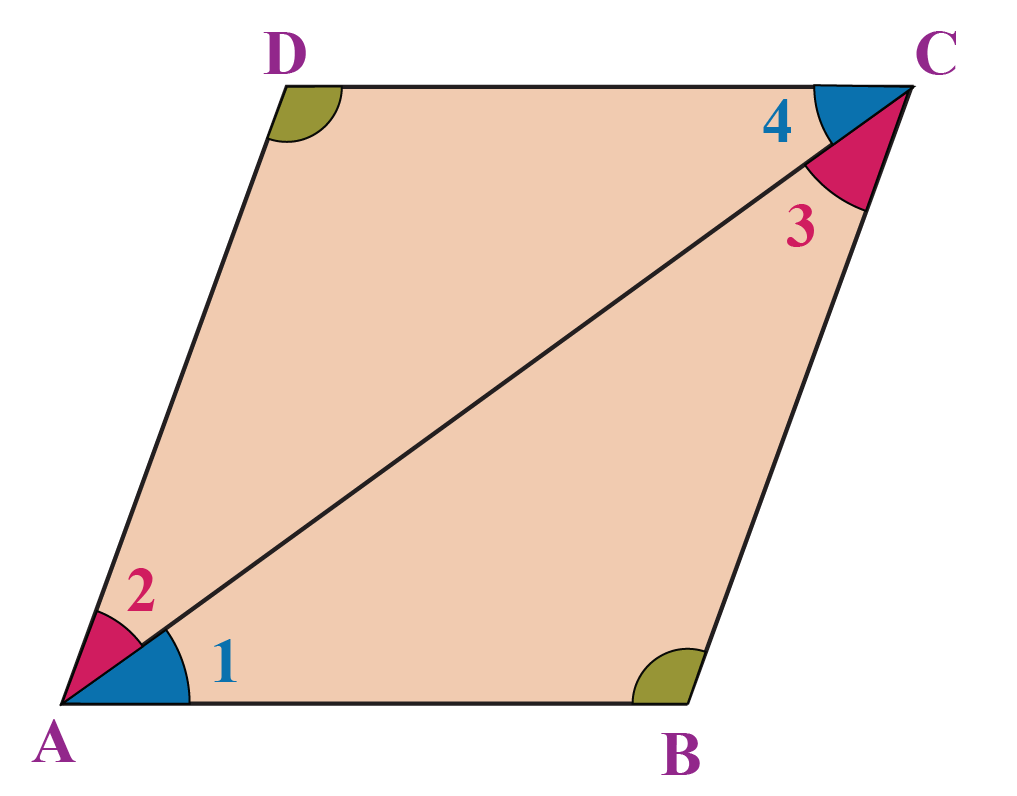### Proof:

First, we assume that $$ABCD$$ is a parallelogram.

Compare $$\Delta ABC$$ and $$\Delta CDA$$ once again:

\begin{align} & AC=AC \\ &\left( \text{common sides}\right) \\\\ & \angle 1=\angle 4 \\ &\left( \text{alternate interior angles} \right) \\\\ & \angle 2=\angle 3 \\ &\left( \text{alternate interior angles} \right) \end{align}

Thus, the two triangles are congruent, which means that

\begin{align}\boxed{\angle B=\angle D} \end{align}

Similarly, we can show that

\begin{align}\boxed{\angle A=\angle C} \end{align}

This proves that opposite angles in any parallelogram are equal.

Now, we prove the converse of this.

### Converse of the Theorem

If the opposite angles in a quadrilateral are equal, then it is a parallelogram.

Assume that $$\angle A$$ = $$\angle C$$ and $$\angle B$$ = $$\angle D$$.

We have to prove that $$ABCD$$ is a parallelogram.

Consider the following figure: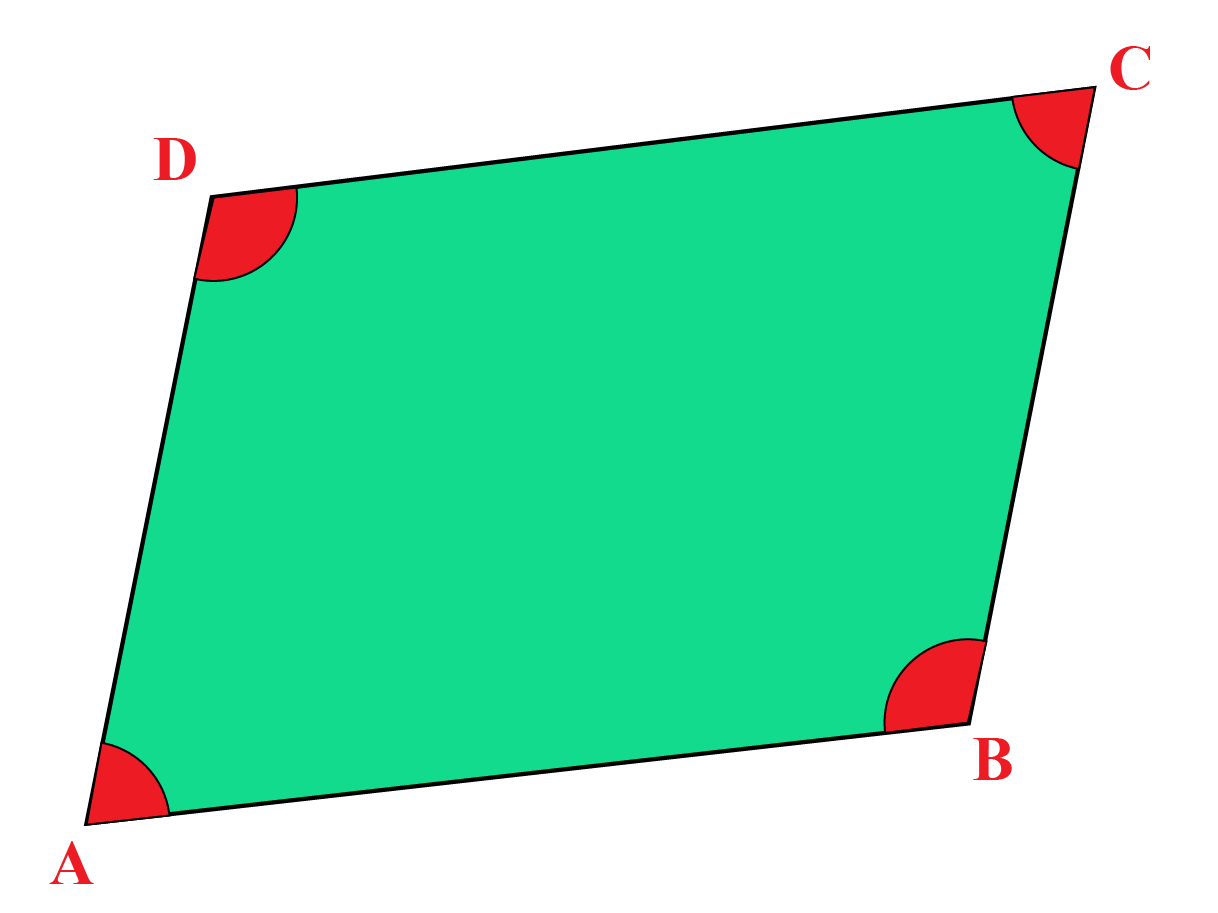We have:

\begin{align}\angle A + \angle B + \angle C + \angle D = \,360^\circ\\2(\angle A + \angle B) =\, 360^\circ\\\angle A + \angle B = \,180^\circ\end{align}

This must mean that $$AD\parallel BC$$

Similarly, we can show that $$AB\parallel CD$$

Hence,

\begin{align}\boxed{ AD\parallel BC\;\text{and}\;AB\parallel CD}\end{align}

and thus, $$ABCD$$ is a parallelogram.

Note that the relation between two lines intersected by a transversal, when the angles on the same side of the transversal are supplementary, are parallel to each other.

## Diagonals of a Parallelogram Bisect Each Other

In a parallelogram, the diagonals bisect each other.

Conversely, if the diagonals in a quadrilateral bisect each other, then it is a parallelogram.

Consider the following figure: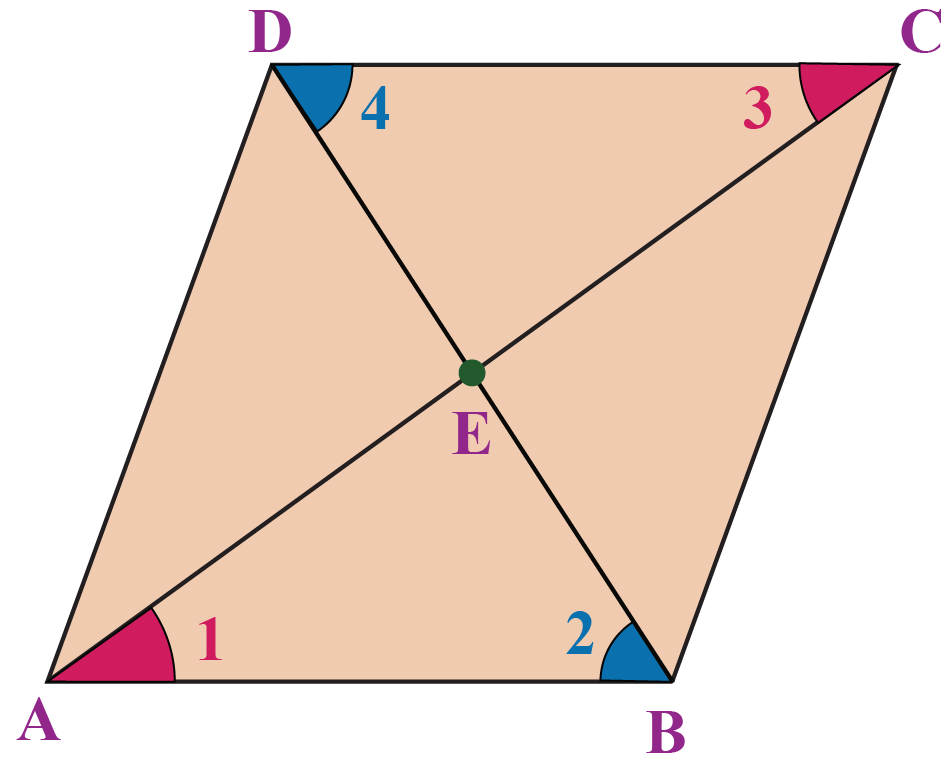### Proof:

First, let us assume that $$ABCD$$ is a parallelogram.

Compare $$\Delta AEB$$ and $$\Delta DEC$$, we have:

\begin{align} & AB=CD \\ &\left( \text{opposite sides of a parallelogram}\right)\\\\ & \angle 1=\angle 3\\ &\left( \text{alternate interior angles}\right) \\\\ & \angle 2=\angle 4\\ &\left( \text{alternate interior angles}\right) \end{align}

By the ASA criterion, the two triangles are congruent, which means that

\begin{align}\boxed{AE=EC\;\text{and}\;BE=ED}\end{align}

Thus, the two diagonals bisect each other.

Now, we will prove the converse of this.

### Converse of  the Theorem

If the diagonals in a quadrilateral bisect each other, then it is a parallelogram

Suppose that the diagonals AC and BD bisect each other.

Compare $$\Delta AEB$$ and $$\Delta DEC$$ once again. We have:

\begin{align} & AE=EC \\ &\left( \text{given}\right) \\\\ & BE=ED \\ &\left( \text{given}\right) \\\\ & \angle AEB=\angle DEC\\ &\left( \text{vertically opposite angles}\right) \end{align}

By the SAS criterion, the two triangles are congruent, which means that:

$$\angle 1$$ = $$\angle 3$$

$$\angle 2$$ = $$\angle 4$$

Hence,

\begin{align}\boxed{AB\parallel CD\;{\rm{and}}\;AD\parallel BC} \end{align}

Thus, $$ABCD$$ is a parallelogram.

## One Pair of Opposite Sides is Equal and Parallel in a Parallelogram

Consider the following figure: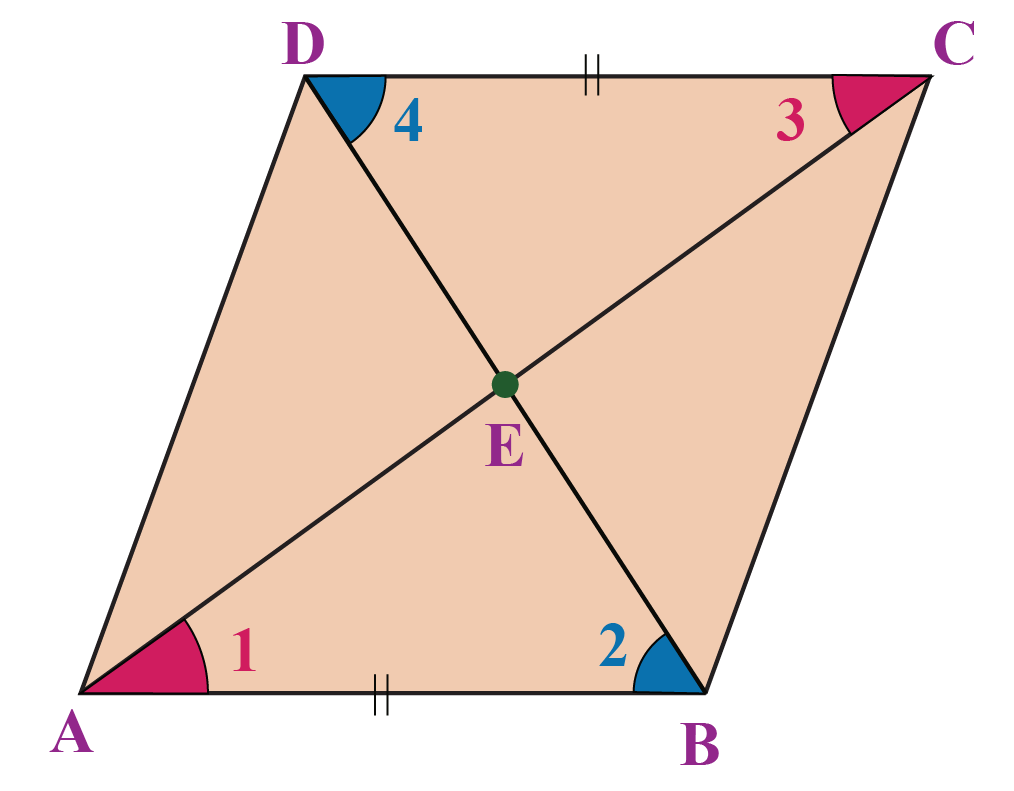It is given that $$AB=CD$$ and $$AB || CD$$

We have to prove that $$ABCD$$ is a parallelogram.

### Proof:

Compare $$\Delta AEB$$ and $$\Delta DEC$$. We have:

\begin{align} & AB=CD\\ &\left( \text{given}\right)\\\\ & \angle 1=\angle 3 \\ &\left( \text{alternate interior angles}\right)\\\\ & \angle 2=\angle 4\\ &\left( \text{alternate interior angles}\right) \end{align}

Thus, the two triangles are congruent, which means that

\begin{align}\boxed{AE=EC\;\text{and}\;BE=ED}\end{align}

Therefore,the diagonals AC and BD bisect each other, and this further means that $$ABCD$$ is a parallelogram.

## Solved Examples

 Example 1

If one angle of a parallelogram is 90o, show that all its angles will be equal to 90o.

Solution:

In any parallelogram, the alternate angles are equal and the adjacent angles are supplementary.

Consider the parallelogram $$ABCD$$ in the following figure, in which $$\angle A$$ is a right angle: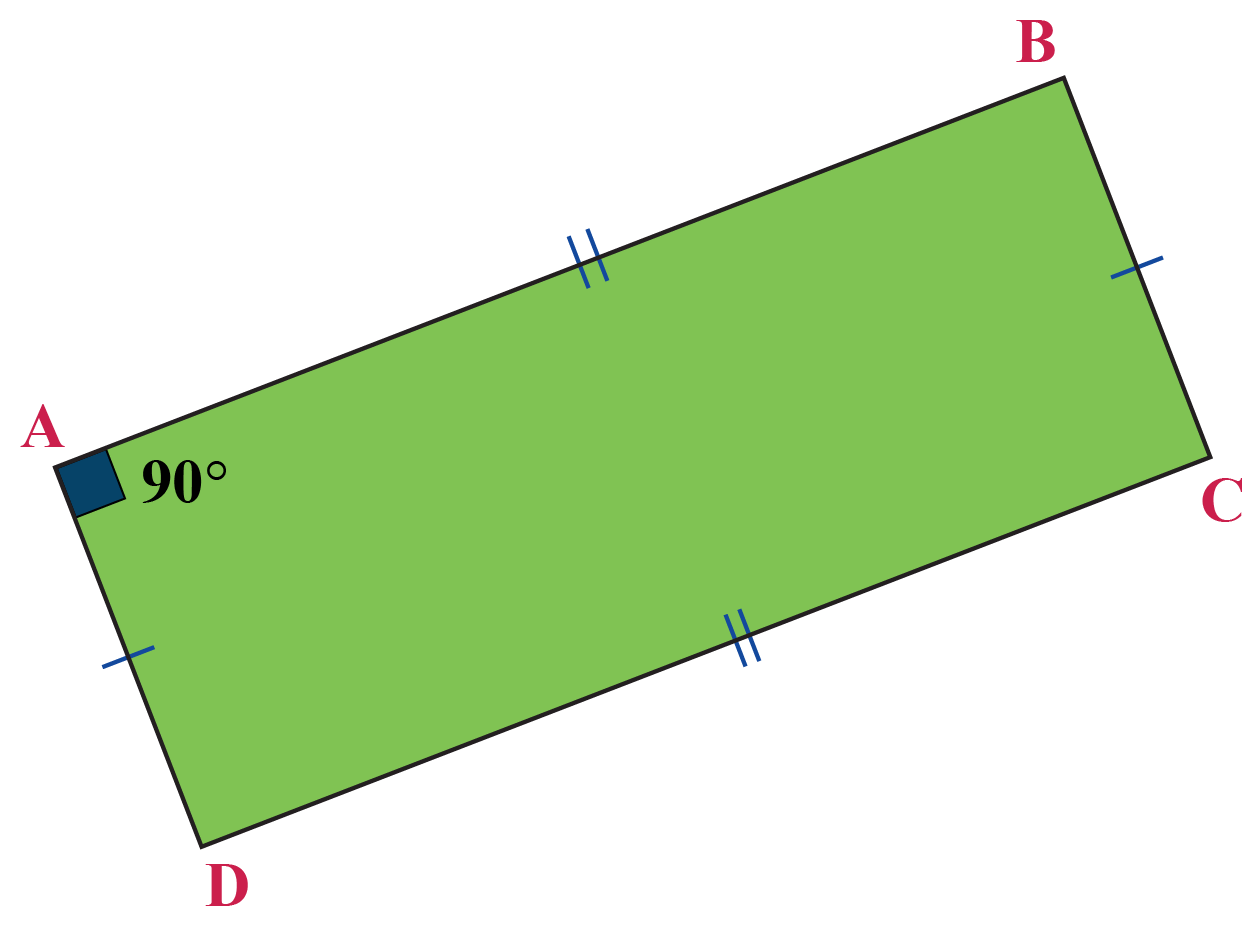Thus, $$\angle C$$ must also be 90o, while

\begin{align}\angle B = \angle D &=\,180^\circ - \;90^\circ \\\\&=\,90^\circ\end{align}

Hence,

\begin{align}\boxed{\angle A=\angle B=\angle C=\angle D = 90^\circ} \end{align}

Clearly, all the angles in this parallelogram (which is actually a rectangle) are equal to 90o.

 $$\therefore$$ when one angle is 90o, the parallelogram is a rectangle
 Example 2

If the diagonals of a parallelogram are equal, then show that it is a rectangle.

Solution:

Consider a parallelogram $$ABCD$$ with digonals $$AC$$ and $$BD$$.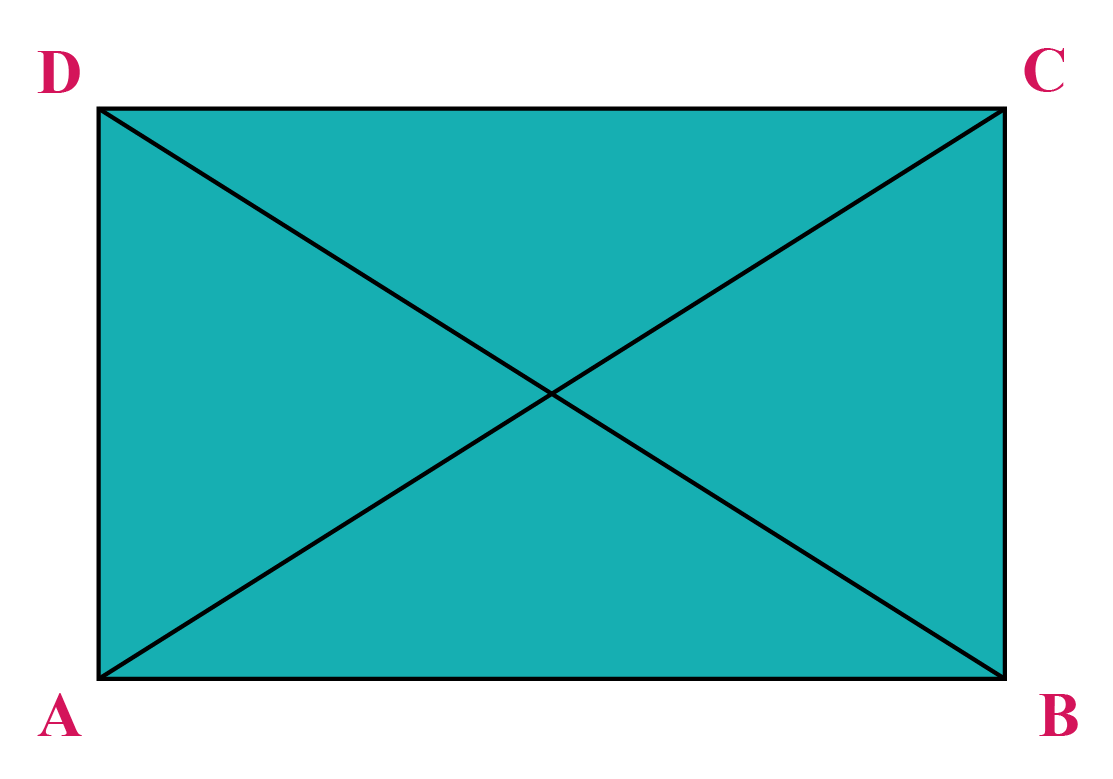Given:

• $$ABCD$$ is a parallelogram
• $$AC$$ = $$BD$$

To prove: $$ABCD$$ is a rectangle.

In $$\Delta ABC$$  and  $$\Delta ABD$$,

• $$AB = AB$$ (common side)
• $$AC = BD$$ (given)
• $$BC = AD$$ (opposite sides of a parallelogram are equal)

Therefore,

$$\Delta ABC \cong \Delta BAD$$

[ by SSS congruence axiom]

By CPCT,

$$\angle ABC = \angle BAD$$ -------- (1)

But also,

$$\angle ABC + \angle BAD$$ = 180°

(co - interior angles are supplementary)

$$\implies$$ $$\angle ABC$$ + $$\angle ABC$$ = 180° (from (1))

Therefore,

$$\angle ABC$$ = 90°

$$\implies$$ All angles are 90° (corresponding angles are equal and consecutive angles are supplementary in a parallelogram)

 $$\therefore$$ ABCD is a rectangle
 Example 3

What is the difference between the opposite angles of a parallelogram?

a) 180°
b) 90°
c) 0°
d) 360°

Solution:

In a parallelogram, the opposite sides and opposite angles are equal.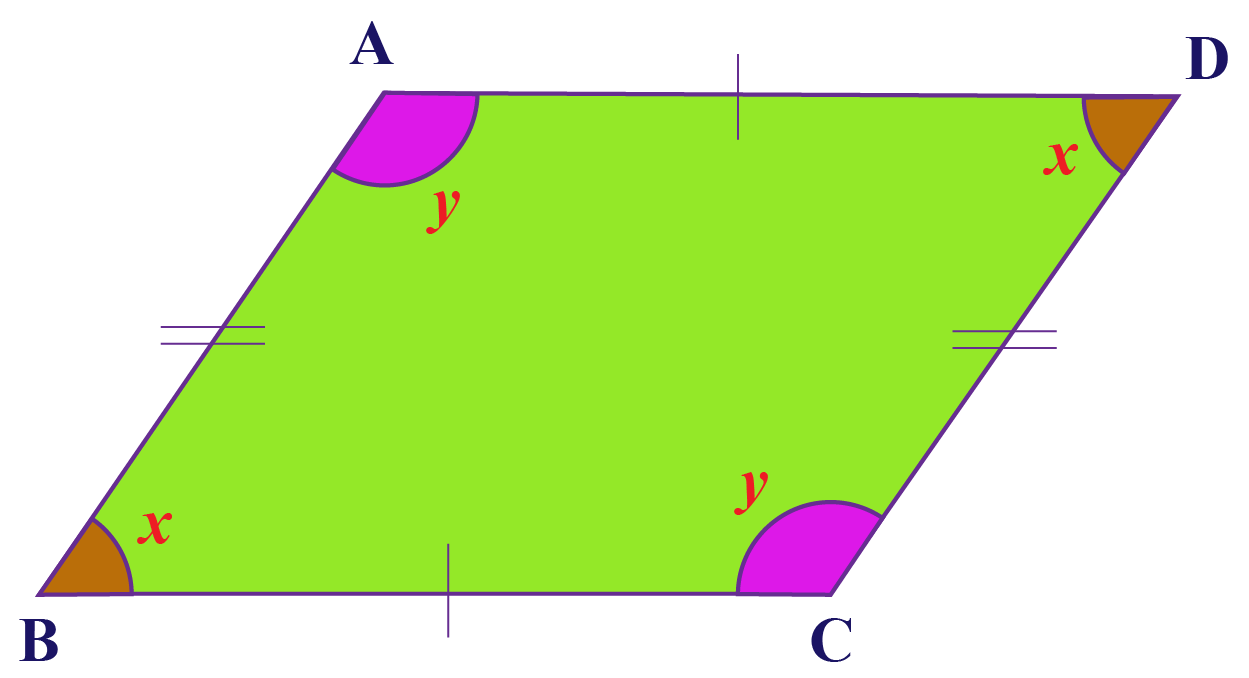Therefore, the difference between the opposite angles of a parallelogram is:

$$x-x = 0^\circ$$

$$y-y = 0^\circ$$

 $$\therefore$$ Difference between opposite angles of a parallelogram is 0°
 Example 4

In a quadrilateral $$ABCD$$, the diagonals $$AC$$ and $$BD$$ bisect each other at right angles.

Show that the quadrilateral is a rhombus.

Solution:

Consider the following figure: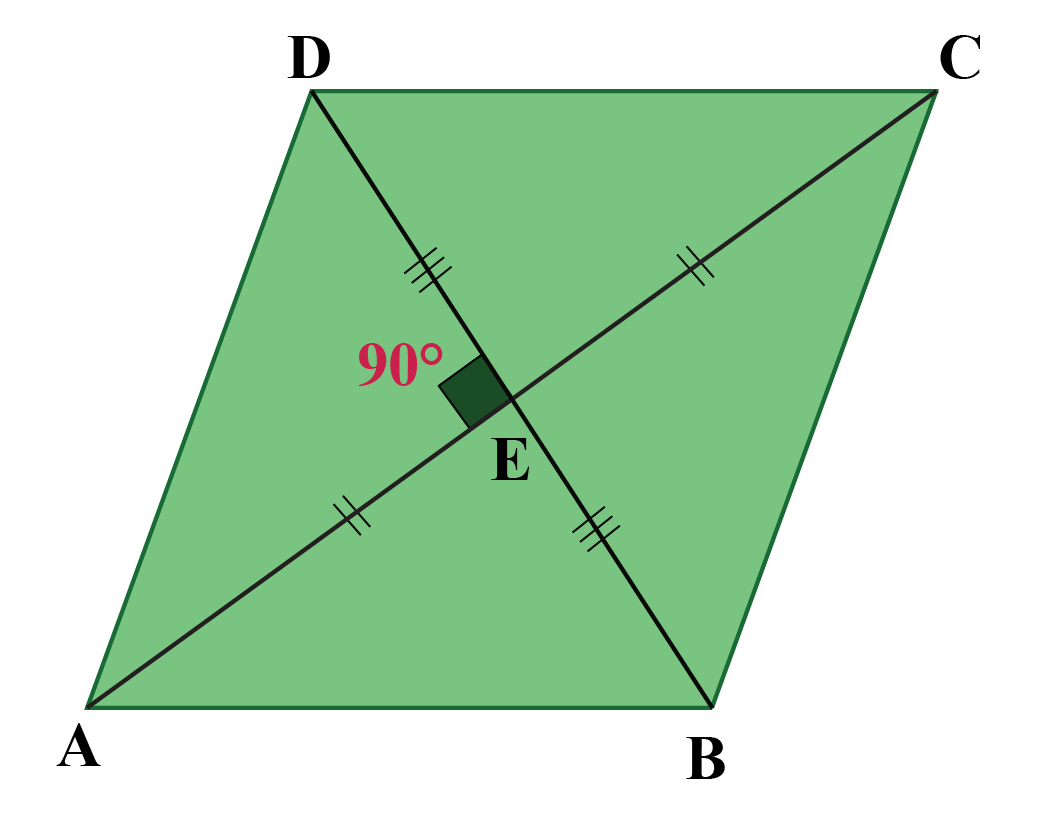First of all, we note that since the diagonals bisect each other, we can conclude that $$ABCD$$ is a parallelogram.

Now, let us compare $$\Delta AEB$$ and $$\Delta AED$$:

\begin{align} & AE=AE\ \ \ \ \\&\left( \text{common}\right) \\\\ & BE=ED\ \ \ \ \ \ \\&\left( \text{given}\right) \\\\ & \angle AEB=\angle AED=\,90^\circ\ \ \ \ \ \ \\&\left( \text{given}\right) \end{align}

Thus, by the SAS criterion, the two triangles are congruent, which means that

$$AB$$ = $$CD$$

This further means that

\begin{align}\boxed{ AB=BC=CD=AD} \end{align}

Clearly, $$ABCD$$ is a rhombus.

 $$\therefore$$ Parallelogram ABCD is a rhombus
 Example 5

$$ABCD$$ is a quadrilateral in which the diagonals bisect each other.

Show that $$B$$ and $$D$$ are equidistant from $$AC$$.

Solution:

Since its diagonals bisect each other, $$ABCD$$ is a parallelogram.

Consider the following figure: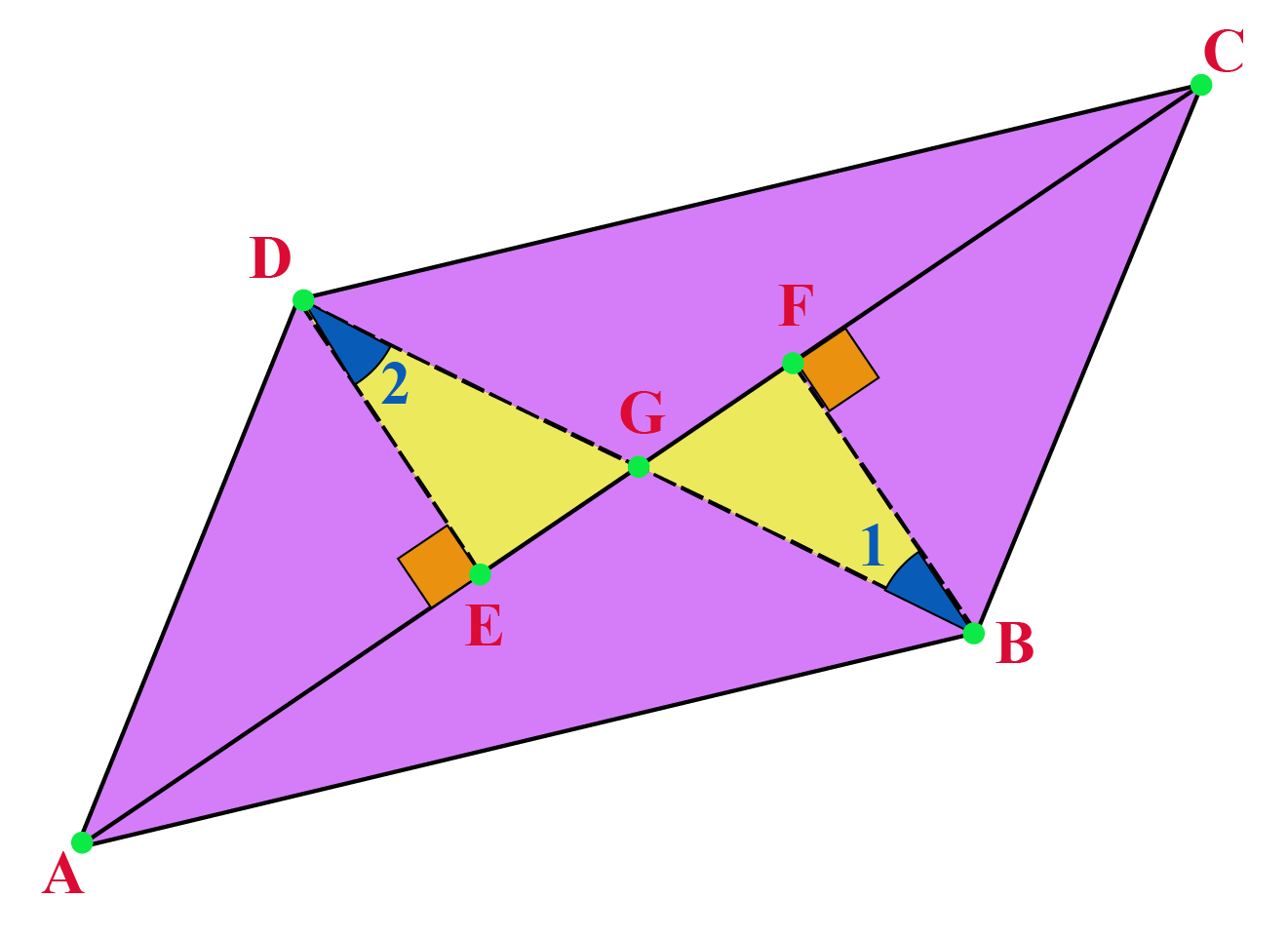Essentially, we have to show that

$$BF$$ = $$DE$$

Compare $$\Delta BFG$$ with $$\Delta DEG$$.

\begin{align} & BG=GD\ \ \ \ \\&\left( \text{diagonals bisect each other}\right) \\\\ & \angle BGF=\angle DGE\ \ \ \ \ \ \\&\left( \text{vertically opposite angles}\right) \\\\ & \angle 1=\angle 2\ \ \ \ \ \ \\&\left( \text{alternate interior angles}\right) \end{align}

By the ASA criterion, the two triangles are congruent, which means that:

\begin{align}\boxed{ BF=DE} \end{align}

Thus, $$B$$ and $$D$$ are equidistant from $$A$$.

Note: Two lines which are perpendicular to the same line are parallel to each other.

 $$\therefore$$ B and D are equidistant from AC
 Example 6

If in a quadrilateral $$ABCD$$, $$AC$$ bisects angles A and C, show that $$AC$$ is perpendicular to $$BD$$

Solution:

Consider the following figure: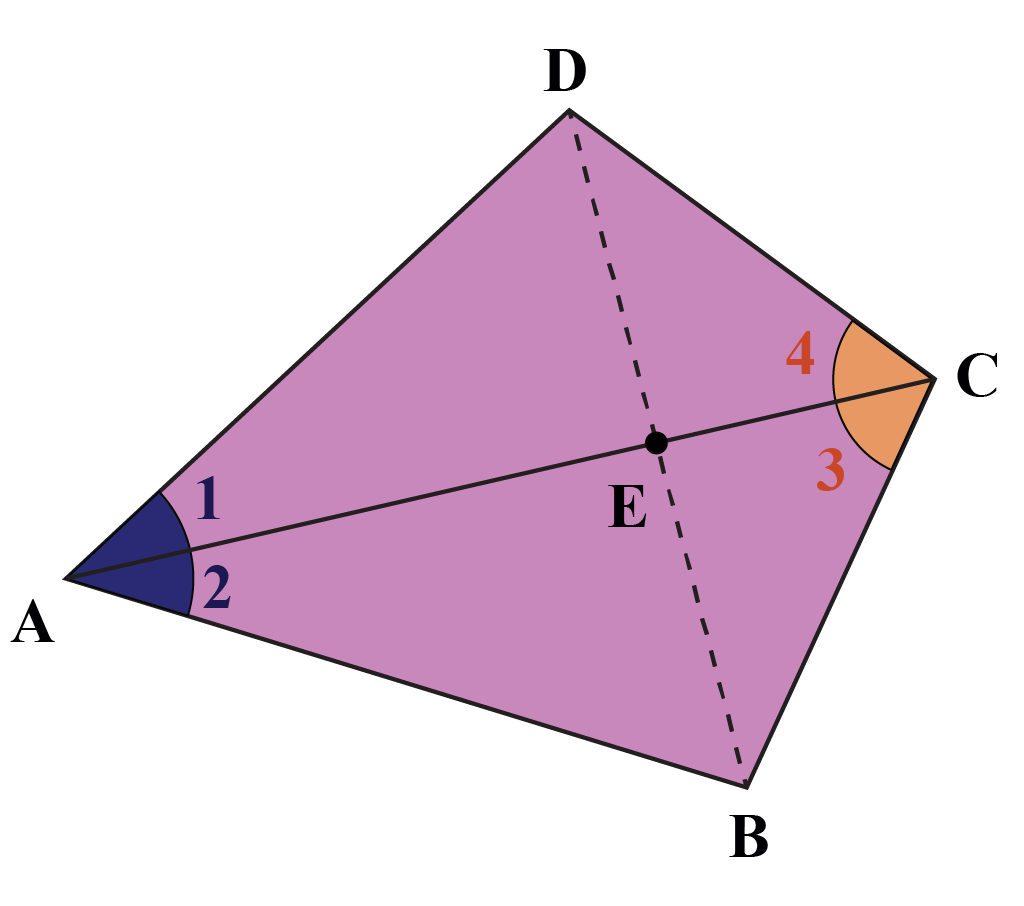Compare $$\Delta ABC$$ and $$\Delta ADC$$:

\begin{align} & AC=AC\ \ \ \ \\&\left( \text{common}\right) \\\\ & \angle 1=\angle 2\ \ \ \ \ \ \\&\left( \text{given}\right) \\\\ & \angle 3=\angle 4\ \ \ \ \ \ \\&\left( \text{given}\right) \end{align}

By the ASA criterion, the two triangles are congruent.

This means that:

$$AB$$ = $$AD$$

Now, compare $$\Delta AEB$$ and $$\Delta AED$$:

\begin{align} & AE=AE\ \ \ \ \\&\left( \text{common}\right) \\\\ & AB=AD\ \ \ \ \ \ \\&\left( \text{shown above}\right) \\\\ & \angle 2=\angle 1\ \ \ \ \ \ \\&\left( \text{given}\right) \end{align}

By the SAS criterion, the two triangles are congruent. Clearly,

\begin{align}\angle AEB = &\angle AED\\\\= &\frac{1}{2} \times 180^\circ \\ \\= &\,90^\circ\end{align}

Hence,

\begin{align}\boxed{\angle AEB=\angle AED = 90^\circ} \end{align}

 $$\therefore$$ AC is perpendicular to BD
 Example 7

Prove that the bisectors of the angles in a parallelogram form a rectangle.

Solution:

Consider the following figure, in which $$ABCD$$ is a parallelogram, and the dotted lines represent the (four) angle bisectors.

We have to show that $$EFGH$$ is a rectangle: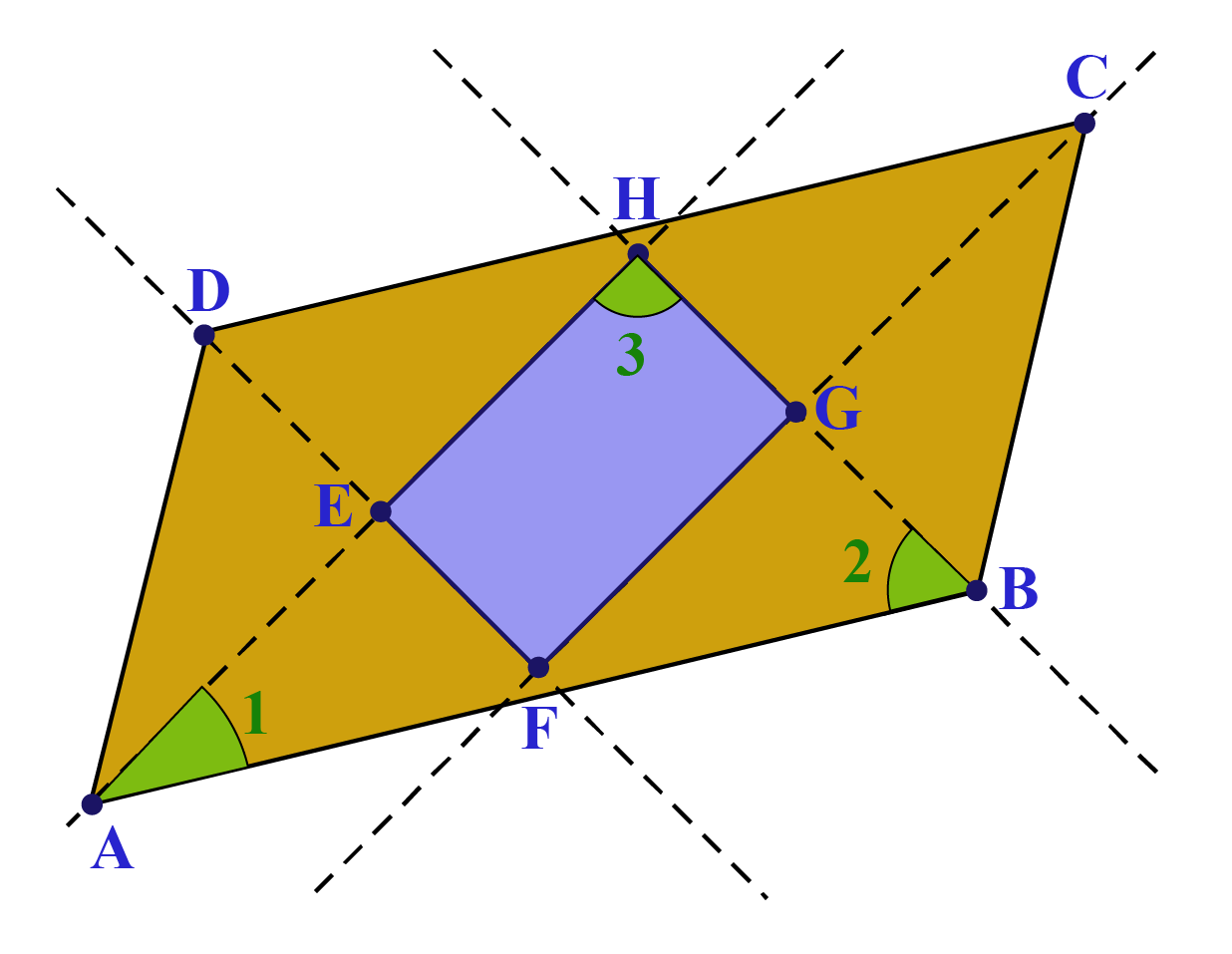We can show this by proving that each of the four angles of $$EFGH$$ is a right angle.

In parallelogram $$ABCD$$, we have:

$$\angle A + \angle B = 180^\circ$$

Now, consider $$\Delta AHB$$.

We have:

\begin{align}\angle 1 + \angle 2 =& \frac{1}{2}\left( {\angle A + \angle B} \right)\\\\ =&\,\ 90^\circ\end{align}

Thus,

\begin{align}\boxed{\angle 3 = 90^\circ} \end{align}

Similarly, we can prove that each of the other three angles of quadrilateral $$EFGH$$ is a right angle.

This means that $$EFGH$$ is a rectangle.

 $$\therefore$$ Bisectors of the angles in a parallelogram form a rectangle
 Example 8

ABC is an isosceles triangle in which AB = AC.

AD bisects exterior angle PAC and CD is parallel to AB, as shown below: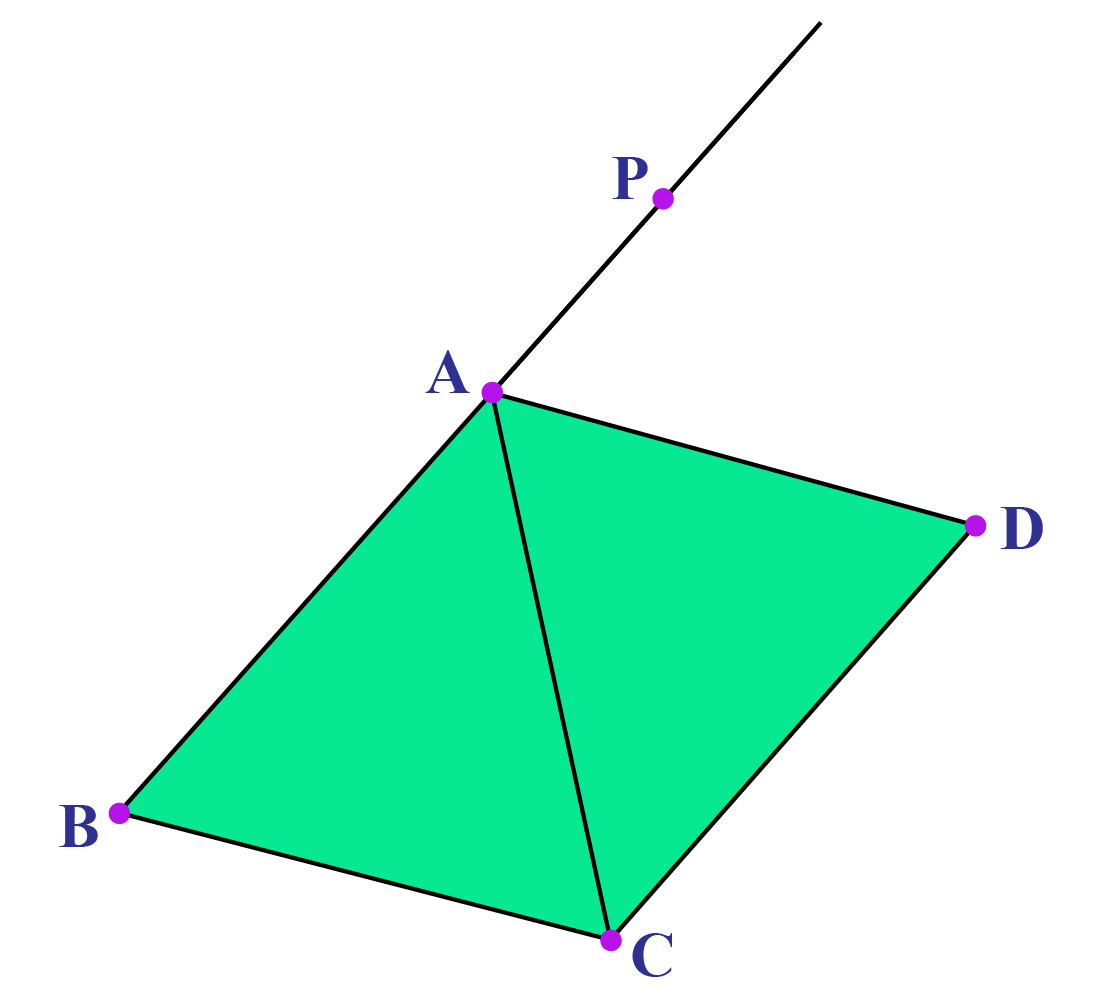Show that:

1. $$\angle DAC$$ = $$\angle BCA$$

2. ABCD is a parallelogram

Solution:

We note that since $$\Delta ABC$$ is isosceles (with $$AB = AC$$), $$\angle B$$ = $$\angle BCA$$.

Now, by the exterior angle theorem,

\begin{align}\angle PAC &= \angle BCA + \angle B \\\\&=2\angle BCA\end{align}

\begin{align}\frac{1}{2}\angle PAC &= \angle DAC \\ \\&= \angle BCA\end{align}

Therefore,

\begin{align}\boxed{ \angle DAC = \angle BCA} \end{align}

Hence,

$$BC$$ = $$AD$$

Combining this with the fact given to us that

$$CD$$ = $$AB$$

Hence,

\begin{align}\boxed{BC\parallel AD\;{\rm{and}}\;CD\parallel AB} \end{align}

Thus, we conclude that ABCD is a parallelogram.

 $$\therefore$$ ABCD is a parallelogram.
 Example 9

Two parallel lines are intersected by a transversal.

Consider the quadrilateral ABCD formed by the bisectors of the interior angles, as shown below: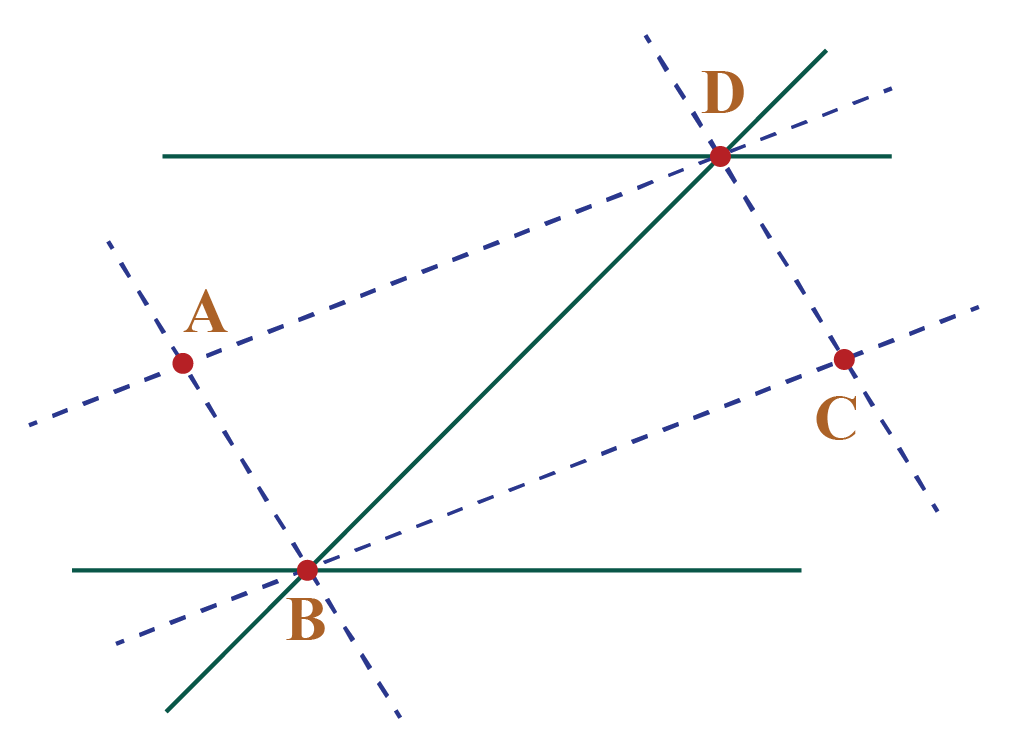Show that ABCD is a rectangle.

Solution:

We first note that the two bisectors of any pair of lines are perpendicular to each other (if you are unsure about this, take a moment to prove it). Thus,

$$AB \bot BC{\rm{\;\;and\;\; }}AD \bot DC$$

Next, we note the following:

\begin{align}\angle ABD + \angle ADB &= \frac{1}{2} \times 180^\circ\,\,\text{(Why?)}\\ \\&=\, 90^\circ\end{align}

\begin{align}\boxed{\angle BAD = 90^\circ} \end{align}

Similarly,

\begin{align}\boxed{\angle BCD = 90^\circ} \end{align}

Thus, we have proved that all the four angles of the quadrilateral ABCD are right angles, which means that ABCD is a rectangle.

 $$\therefore$$ ABCD is a rectangleThink Tank
1. Why is a kIte not a parallelogram?
2. Is an isosceles trapezoid a parallelogram?

IMO (International Maths Olympiad) is a competitive exam in Mathematics conducted annually for school students. It encourages children to develop their math solving skills from a competition perspective.

## 1. What are the 7 properties of a parallelogram?

The 7 properties of a parallelogram are:

1. The opposite sides of a parallelogram are equal.
2. If the opposite sides of a quadrilateral are equal, it is a parallelogram.
3. The opposite angles of a parallelogram are equal.
4. If the opposite angles of a quadrilateral are equal, it is a parallelogram.
5. The diagonals of a parallelogram bisect each other.
6. If the diagonals of a quadrilateral bisect each other, it is a parallelogram.
7. If one pair of opposite sides of a quadrilateral is equal and parallel, then the quadrilateral is a parallelogram.

## 2. What are the properties of the diagonals of a parallelogram?

The properties of the diagonals of a parallelogram are:

• A diagonal of a parallelogram divides it into two congruent triangles.
• The diagonals of a parallelogram bisect each other.

Class 7-8 Important Formulae
Class 7-8 Important Formulae
Class 7-8 Sample Papers
Class 7-8 Sample Papers
Class 9 Important Formulae
Class 9 Important Formulae